# Solution of the Schrödinger Equation for a Simple Harmonic Oscillator

Mohammad Yasir is a physics graduate from the University of Delhi and currently enrolled in the master's programme at IIT.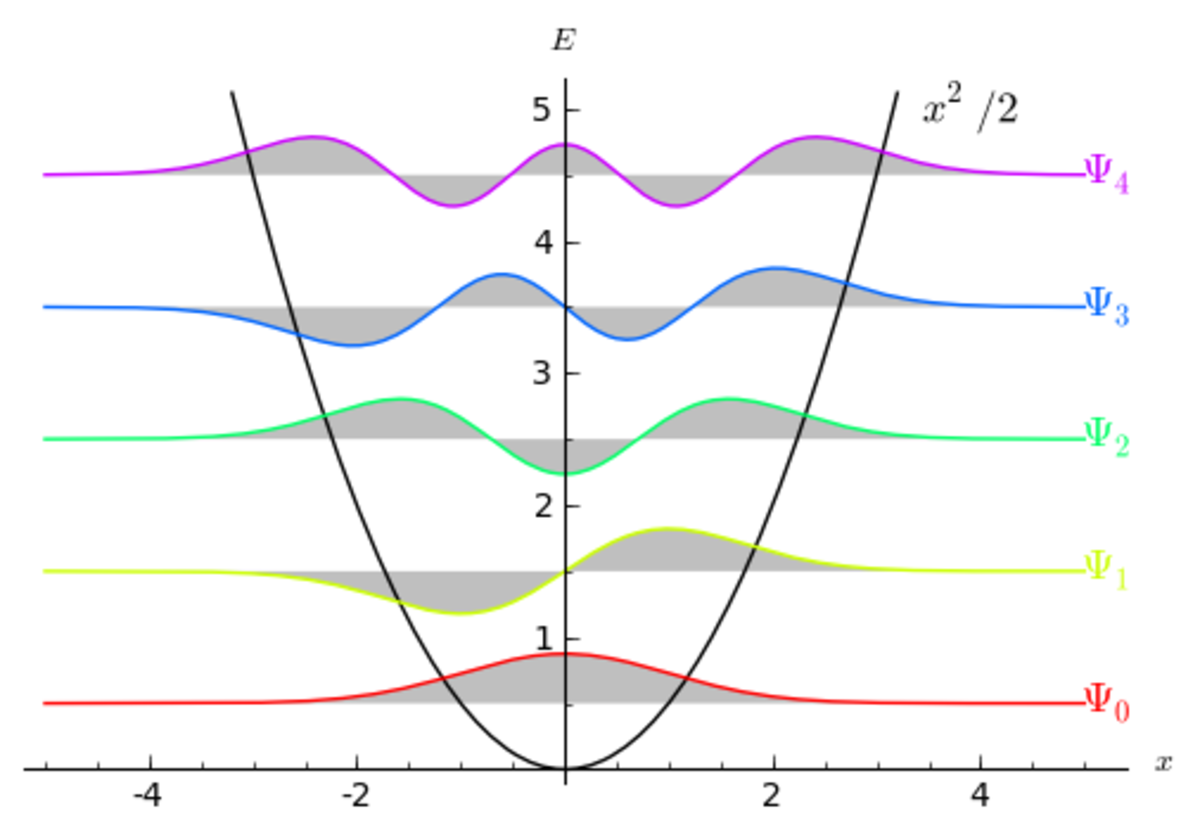Energy Levels of a quantum harmonic oscillator.

## Setting Up the Problem

The Simple Harmonic Oscillator is an excellent study for understanding the concepts of quantum mechanics and Schrödinger equation. It also serves as a fantastic way of seeing the quantum realm morph into the world of classical mechanics as we go to states of higher and higher energy, thereby increasing the value of n.

To begin, recall that SHM is characterized by the equation of motion given as F = -kx. This corresponds to the potential V = ½kx2. The value of the proportionality constant is given by k = mw2. This gives us all the tools we need to set up the Schrödinger equation.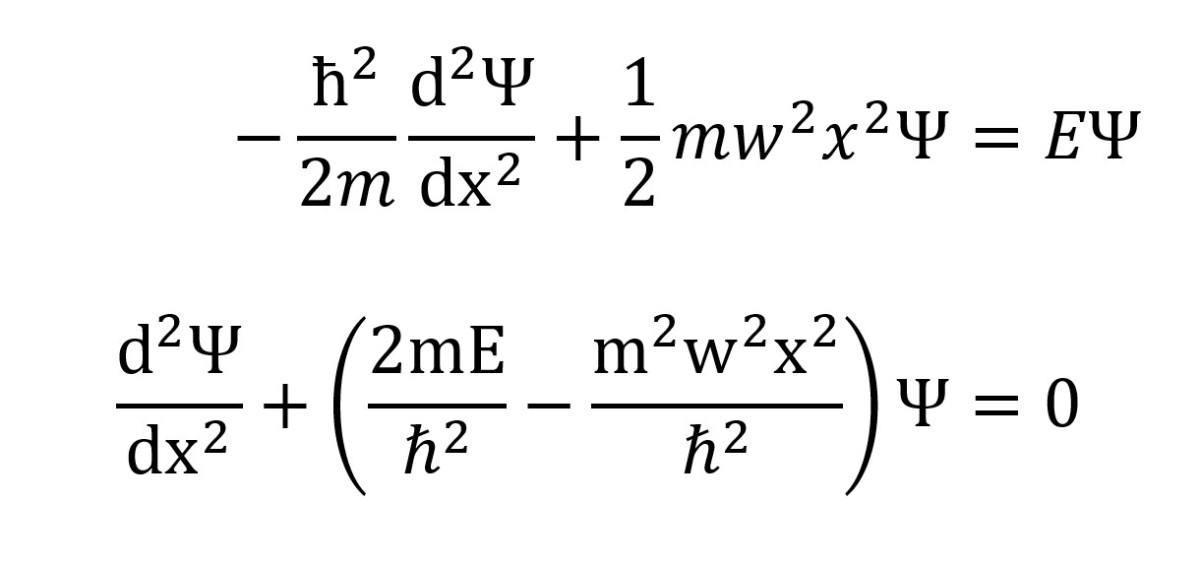With slight rearrangement, Schrodinger's equation for the simple harmonic oscillator takes the above form.

## Solving the Equation

Before we get started, it would prove helpful for us to introduce a slight change of variables and bundle the constants up together.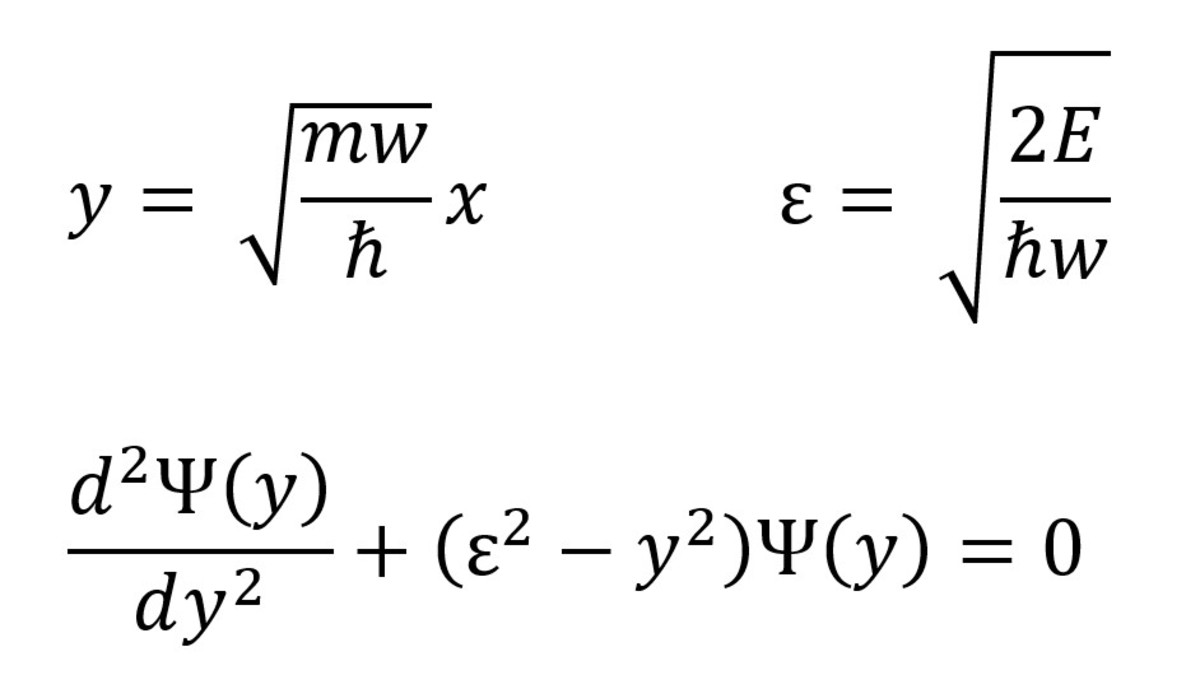EQUATION 1: Arrived at using a change in variables.

As you can see, this little rearrangement significantly cleans up our equation. Now, we first try to solve the asymptotic condition where y approaches infinity and thus, compared to y2, ε2 can be ignored. This allows us to assume a solution in the following form:

## ψ(y) = u(y) exp(-y2/2)

Note that including the additional function u(y) is necessary since we are currently talking about a solution near the asymptotic region. The form and value of u(y) will be calculated later. Further, it will be prudent to know that this value of Ψ(y) wasn't chosen on random. In fact, it makes perfect sense. Take a look:

We now have an expression for the second derivative of Ψ(y) with respect to y. If we put this into equation 1, we will find that the exponential terms cancel out and we are left with an expression purely in terms of u(y). Try it out yourself before proceeding further. You should have an equation that looks like this:

## d2u/dy2 - (2y) du/dy + (ε2 - 1) u = 0 ----- (2)

Can you guess the next step in our process? This equation looks simple enough, but it does not have a direct solution. Instead, we will utilize the power series method to arrive at an expression for u(y). I will not go into details about it since you must have studied it already. If not, I recommend that you go through this pdf, which explains all that you might need to know about power series and solving second order linear ODEs.

We'll now jump to the recurrence relation for u(y), obtained after putting its power series form expression in equation 2.

## Looking at the Power Series

For exceedingly large values of n, the ratio of two successive terms approaches the value 2/n, which diverges much faster than the exponential decay term in our initial solution for ψ(y). Thus, this series must terminate for the wavefunction to hold any physical meaning.

For that to happen, the coefficient with the index n+2 must vanish at a particular value of n, let us say j.

Scroll to Continue

## Read More From Owlcation

Therefore, we have an expression for the maximum possible value of n, represented by j and given by 2j + 1 = ε2

Before proceeding further, recall that ε is related to the energy of our harmonic oscillator and the above equation just gave us an expression for En.

## En = (n + ½) ħw

This leads us to another revolutionary concept.

## What About the Wavefunction?

If you look back, we have technically solved the Schrodinger equation. We know the recurrence relation for the power series form of u(y), and we already know that the complete solution looks like Ψ(y) = u(y) exp(-y2/2). All that remains is to normalize this wavefunction and replace all the variables we changed. Once you do that, you will arrive at an expression that looks like this: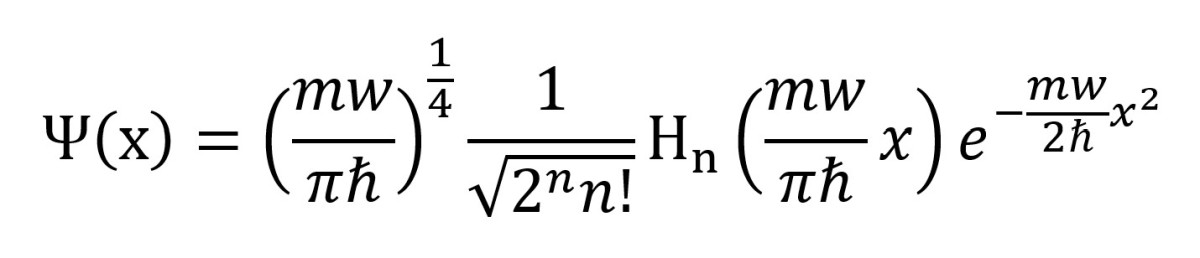The final solution to the harmonic oscillator problem.

The function Hn(x) is what is known as the Hermite Polynomial. I encourage you to learn more about them since you will encounter these polynomials in various physical situations.

## Quantum Tunneling

The image attached in the beginning of this article shows the form our solution takes for the first few states of the simple harmonic oscillator. Note that you can multiply Ψ with complex conjugate Ψ* to obtain an expression for the probability of finding the oscillator at a point x. While I will not discuss it in detail, you can see that the wavefunction does not vanish outside the classically allowed region given by the potential curve. Instead, it decays off. Can you guess how this is happening?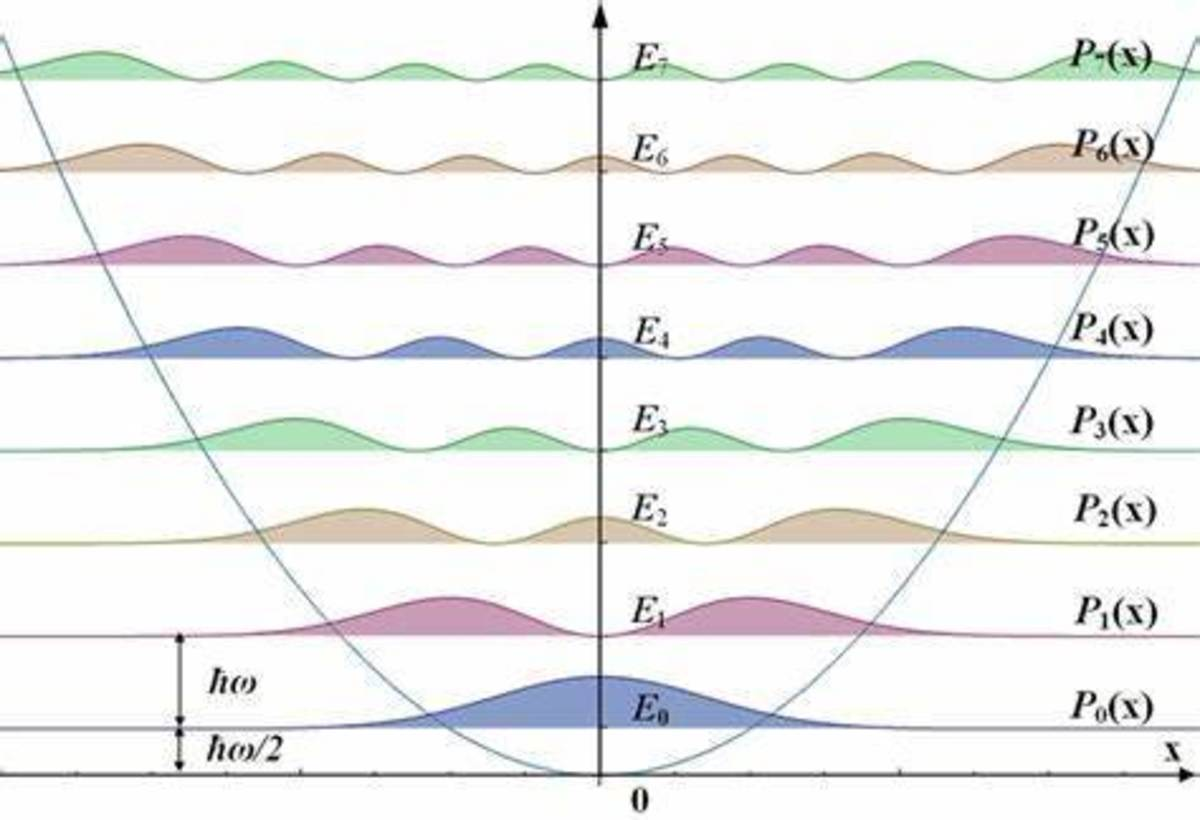The probability curve for a harmonic oscillator and the quantization of energy. The parabolic curve represents potential.

## What Exactly Does Quantization of Energy Mean?

Classically, the energy of a harmonic oscillator is given by E = ½mw2a2, where a is the amplitude of the oscillations. As is evident, this can take any positive value. On the other hand, the expression for the energy of a quantum oscillator is indexed and given by, En = (n + ½)ħw. This leads to two realizations:

1. A quantum oscillator can only exist in certain states, with each of the state characterized by an energy that is a half-integral multiple of ħw. Any energy value apart from these quantized values is not allowed.
2. The ground state energy of a quantum oscillator is not zero, whereas the ground state energy of a classical oscillator is zero.

## Conclusion

This was a basic discussion of what the quantum oscillator problem looks like. The concept is utilized in explaining the electronic properties of metals by considering the electrons to be coupled harmonic oscillators. Various other applications for this phenomenon exist as well.

If you are a university student, I recommend that you follow this link for a few solved harmonic oscillator problems. Further, get your hands on a graphing software and try to graph the wavefunction for n = 10. Try again for n = 100. Compare the curve you obtain with the classical probability density. What inferences can you draw from it?

This content is accurate and true to the best of the author’s knowledge and is not meant to substitute for formal and individualized advice from a qualified professional.